﻿ New Modeling Approach for Engine Cold Testing Driveline Torsional Vibration Analysis with Increased AccuracyPublications are Open
Access in this journal
Article Versions
Export Article
• Normal Style
• MLA Style
• APA Style
• Chicago Style
Research Article
Open Access Peer-reviewed

### New Modeling Approach for Engine Cold Testing Driveline Torsional Vibration Analysis with Increased Accuracy

Ra’uf Tailony
American Journal of Mechanical Engineering. 2017, 5(4), 156-160. DOI: 10.12691/ajme-5-4-7
Published online: July 19, 2017

### Abstract

Internal combustion engine cold test is becoming one of the main tests performed during the late stage of the product development for defects inspection using the torque signatures and pressure levels in various engine components. This paper includes a lumped masses model generated for the cold test torsional system to calculate the driveline natural frequencies with a high accuracy. Calculating the natural frequency of the system is crucial for avoiding resonance excitation during the testing phase. Eigen value problem was solved; the natural frequencies and the mode shapes were obtained. The natural frequencies of the model were compared with the measured natural frequencies and 95% accuracy is achieved.

### 1. Introduction

Cold testing of the internal combustion engines is increasingly used to predict the engine defects and flaws without running the engine. Cold test has an advantage on the hot test for the fact that cold test functions without firing up the engine hence requires less hardware by eliminating the fuel pump and fuel lines 1.

Cold test includes a driving electrical motor connected through a driveline that delivers the power to the I.C engine crankshaft and the pistons through the flywheel, as shown in Figure 1. The signals coming from the various engine sensors are analyzed and compared with the simulated reference signals. Each distorted signal represents a certain malfunction in the engine hardware.

• Figure 1. Motor-Driveline-Engine illustration

The torsional vibrations exist in all the machines with rotating parts, these vibrations remain unnoticed while the operating frequency is out of the system’s natural frequency range. If the operating speed reaches the critical speed of the system; a resonance distortion takes place and the signals from various sensory become unusable for different testing procedures 2.

Driveline torsional modeling requires the properties of the drive motor and the engine specifications. Boysal et.al. 8 studied the torsional vibrations for a single cylinder multi-body engine, by analyzing the forces generated inside the cylinder block and their effects on the crankshaft alignment and the engine output torque. Pitchaikani et.al. used a software assisted modeling for a powertrain torsional vibration system to study the NVH behavior of the driveline but no model efficiency measurements were included 9. This paper is filling the gap of measuring the torsional vibrations with a simplified lumped model that is built by making a carefully selected mass equivalency approach which reduce the system’s complexity while keeping the same physical properties as inertia and stiffness for the lumped assemblies and compare the results with the experimental measurements of the torsional system. The measured versus modeled natural frequency values were aligned on a Campbell diagram for a direct critical speeds evaluation. Zhenpeng et.al. performed a modal analysis on a 4 cylinders engine using the component mode synthesis. Although (CMS) is a fast modeling methodology but the accuracy does not exceed 75% 10. In this paper a lumped mass specialization approach was used, and the efficiency of the model was measured for the purpose of converging to the real system properties.

Theoretical vibrational analysis of the drivelines is a useful tool for critical speeds identification, the modeling methodologies explained in the literature couldn’t replace the experimental approach yet 3, 4. In this paper an enhanced approach is explained with an increased TVA accuracy.

### 2. Theoretical Vibrational Analysis

2.1. Mathematical Modeling

Creating a driveline model for a torsional vibrational analysis requires the elastic properties of the driveline components. The simple cold test setup includes an electrical motor, a connecting driveline and a target engine. The main driveline components are the clutch coupling, the torque meter, the main coupling and the spindle, shown in Figure 2.

• Figure 2. Driveline main components

The model is generated by creating a system of lumped inertial masses fixed on a uniform shaft, Figure 3. Each shaft connecting two lumped masses is compensated with a spring that has a torsional stiffness value equal to the lumped mass stiffness, and each lumped mass is assigned with its polar inertial value, shown in Figure 4.

• Figure 3. Lumped masses schematic
• Figure 4. Spring-mass system schematic
2.2. Equations of Motion

The equations of motion can be derived using Newton’s second law: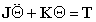(1)

Where J: Polar inertial matrix; K: Torsional stiffness matrix; T: Input torque vector matrix.

Since the model is assumed to be a fixed-free structure, the torque vector matrix is set to zero.

Eq. (1) becomes: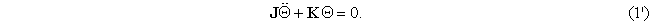By defining the material type of each lumped mass, the torsional stiffness matrix can be obtained, as shown below.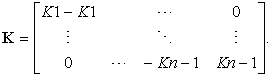(2)

The diagonal inertial matrix is required for finding the system’s natural frequency. Based on the geometry of the driveline, Eq. 3 can be used to find the polar inertia values.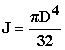(3)

Where D: the diameter of the rotating shaft.

After considering the various sections of the driveline and calculating the polar inertia related to each section; the diagonal polar matrix should be similar to Eq. (4).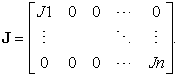(4)
2.3. Eigen Value Problem

To solve the eigen value problem, the torque is assumed to be zero (T=0) 5. The characteristic equation is calculated using the following equation: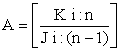(5)

Where i ≥1, 0 < n ≤19.

Solving Eq. (5) for a 19 DOF structure obtains the eigen values and eigen vectors of the system which represent the natural frequencies and mode shapes. Angular speed can be obtained using Eq. (6) shown below.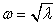(6)

Where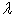: The system’s Eigen value.

Consequently, the natural frequencies of the system are obtained using the following equation.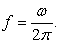(7)

The analytical method is used for solving the equations of motion. Friction parameters are assumed to avoid any singularity in the system e.g. The system structure is assumed to be fixed-free structure. Some material elastic parameters are neglected as the compressive strength, thermal conductivity and material damping ratio. The more parameters assumed in the model the less the accuracy of the calculated natural frequencies.

The model is designed to find the first five natural frequencies of the system since they are satisfactory for predicting the resonance range of the system 6.

2.4. Experimental Procedures

The driveline is powered by Asynchronous electrical motor (Rexroth IndraDyn A, MAD 160C). The driveline is designed to run at 500 rpm speed and 270 N.m torque. A non-contact torque meter (HBM-T40B) is coupled along the driveline to read the torque signatures versus time during the test. On the end of the driveline a 6 cylinders inline engine is mounted through the flywheel. The cold test is performed by passively ramping the engine from 0 to 500 RPM. The natural frequencies of the system were verified by enabling the torque meter to start reading and applying a hammer modal impact on the engine side without rotation and comparing them with the natural frequencies detected in the torque trace generated by the driveline rotation, the torque signatures readings are shown in Figure 5.

• Figure 5. Modal impact torque signature with time

### 3. Results

3.1. Modeling Results

The equations of motion are plugged in MATLAB in a matrix form, the code is written to solve for the first five natural frequencies of the system. The lumped masses system is plugged in simulation Xpress analysis CAD software, Figure 6. The initial conditions and material properties are defined similar to the conditions used in the analytical calculations. The analytical and simulation results are crossed and matched. The final natural frequencies values are shown in Figure 7.

• Figure 6. Modeled lumped masses
• Figure 7. Crossed frequency values (Frequency vs. vibration mode)
3.2. Experimental Results

The experimental results are performed on a cold test stand equipped with a 3.5 Liters, 6 cylinders, 4 strokes research and development gas engine. The driveline consists of a coupling clutch, main coupling, torque meter and a spindle as described previously in Figure 2. The driving electric motor is three phases, 12 HP, 60 Hz, directly bolted to the clutch coupling with no gear to gear meshing.

For detecting and measuring the resonance of the system, 3 industrial type accelerometers were used for frequency amplitudes measurements, with 50g sensitivity capability, one of them was mounted on the engine chassis, the other on the electric motor body and the third on the torque meter mounting. The torque meter data is used for the system stability measurements and detection accuracy, Figure 8.

• Figure 8. Measurement sensors locations

The results of the experimental measurements compared with the modeling outcome are shown in Figure 9. Crossing the obtained natural frequencies of the system with the engine excitation and the driveline speed helps in determining the critical speed of the system, in which the resonance could occur.

By analyzing Figure 9, the critical speed range is found to be 2050 - 2150 RPM. Since the critical speed is in the range of the operating speed, a special attention is needed by monitoring the driveline operating speed to avoid the resonance excitation which could lead to a major failure in the system, or adjusting the system’s total inertia to clear from the resonant speed range 7.

• Figure 9. Campbell diagram for 2nd mode measured and calculated natural frequency

### 4. Conclusions

The Torsional vibrational analysis is important for any rotating assembly, it has to be performed before attempting to run the system. The accuracy of the mathematical modeling depends on the accuracy of the input variables as the torsional stiffness and the polar inertias values. The CAD modeling precision can be increased by properly designing the lumped masses system and mimicking the structure response in the real system. Defining the range of the critical speeds is the goal for the TVA studies, and considered a part of the preventive maintenance and the failure prediction systems.

The results showed a high accuracy for the model mode analysis versus the experimental data. Ignoring the model joining details as the bolts and fittings reduces the modeling time and help in simplifying the modeling process. Friction forces are not a critical parameter in measuring the natural frequencies of the system hence can be neglected in parallel with the electrical drive skidding and the armature coil hesitation.

Campbell diagram included only the excitations generated by the internal combustion engine, a comprehensive Campbell diagram is required with the excitations generated by the electrical drive motor and the lateral driveline vibrations. Including all the possible harmonic excitations help determining more speed areas that may cause the system to resonate, and consequently help the designers determining the stability and durability of their future systems.

### References

  K.A.Gul, D.E.Adams, “Reducing Torsional Vibration In An Engine Cold-Test Cell For Improved Structural Reliability And Engine Assembly Defect Diagnostics,” in ASME Paper, 2009, DSCC 2009. In article View Article  Feese, T. D., “Torsional Vibration Linked to Water Pumping System Failure,” Pumps and System Magazine, 1997 pp. 44-45. In article  D. Siano, R. Citarella, “Elastic Multi Body Simulation of a Multi-Cylinder Engine,” The Open Mechanical Engineering Journal, 2014, 8, 157-169. In article View Article  R. G. Desavale, A. M. Patil, “Theoretical and Experimental Analysis of Torsional and Bending Effect on Four Cylinders Engine Crankshafts by Using Finite Element Approach,” International Journal of Engineering Research, 2013, 2, 379-385. In article  G. Rui, Jang, Choi, “Torsional vibration analysis of lathe spindle system with unbalanced workpiece,” J. Cent. South Univ. Technol., 2011, 18, 171-176. In article View Article  K. A. Gul, Thesis: Modeling and Analysis of Engine Cold-Test Cells for Optimizing Driveline Design for Structural Reliability and Engine Assembly Defect Diagnostics, 2013. In article View Article  G. Genta, “Vibration dynamic and control,” Springer, 2009, pp.764-766. In article View Article  A. Boysal, H. Rahnejat, “Torsional vibration analysis of multi-body single cylinder internal combustion engine model,” Appl. Math. Modelling, 1997, 21, 481-493. In article View Article  A. Pitchaikani, S. Venkataraman, K. K. Koppu, “Powertrain torsional vibration system model development in Modelica for NVH studies,” in Proceedings 7th Modelica Conference, The Modelica Association, 444-453. In article View Article  H. Zenpeng, G. Wenqin, X. Weisong, “NVH and reliability analyses of the engine with different interaction models between the cranckshaft and bearing,” Applied Acoustics, 2016, 101, 185-200. In article View ArticleThis work is licensed under a Creative Commons Attribution 4.0 International License. To view a copy of this license, visit http://creativecommons.org/licenses/by/4.0/